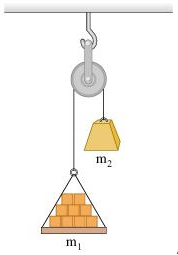# Problem: A load of bricks with mass = 15.0 kg hangs from one end of a rope that passes over a small, frictionless pulley. A counterweight of mass = 27.6 kg is suspended from the other end of the rope, as shown in the figure. The system is released from rest.1) What is the magnitude of the upward acceleration of the load of bricks?2) What is the tension in the rope while the load is moving?

###### FREE Expert Solution

Newton's Second law:

$\overline{){\mathbf{\Sigma }}{\mathbf{F}}{\mathbf{=}}{\mathbf{m}}{\mathbf{a}}}$

1)

$\begin{array}{rcl}\mathbf{T}\mathbf{-}{\mathbf{m}}_{\mathbf{1}}\mathbf{g}& \mathbf{=}& {\mathbf{m}}_{\mathbf{1}}\mathbf{a}\\ \mathbf{T}\mathbf{-}{\mathbf{m}}_{\mathbf{2}}\mathbf{g}& \mathbf{=}& \mathbf{-}{\mathbf{m}}_{\mathbf{2}}\mathbf{a}\end{array}$

Substracting the equations:

90% (84 ratings)###### Problem DetailsA load of bricks with mass = 15.0 kg hangs from one end of a rope that passes over a small, frictionless pulley. A counterweight of mass = 27.6 kg is suspended from the other end of the rope, as shown in the figure. The system is released from rest.

1) What is the magnitude of the upward acceleration of the load of bricks?

2) What is the tension in the rope while the load is moving?